## Ultraspherical Polynomial

The ultraspherical polynomials are solutionsto the Ultraspherical Differential Equation for Integerand. They are generalizations of Legendre Polynomials to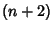-D space and are proportional to (or, depending on the normalization, equal to) the Gegenbauer Polynomials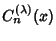, denoted in Mathematica(Wolfram Research, Champaign, IL) GegenbauerC[n,lambda,x]. The ultraspherical polynomials are also Jacobi Polynomials with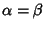. They are given by the Generating Function(1)

and can be given explicitly by(2)
whereis a Jacobi Polynomial (Szegö 1975, p. 80). The first few ultraspherical polynomials are(3)(4)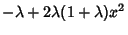(5)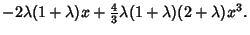(6)

In terms of the Hypergeometric Functions,(7)(8)(9)

They are normalized by(10)

Derivative identities include(11)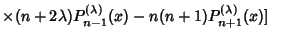(12)(13)(14)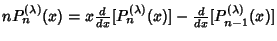(15)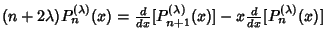(16)(17)(18)
(Szegö 1975, pp. 80-83).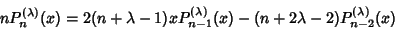(19)

for, 3, ....

Special double-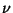Formulas also exist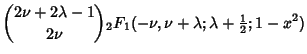(20)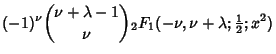(21)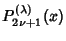(22)(23)

Special values are given in the following table.Special PolynomialLegendre Polynomial 1 Chebyshev Polynomial of the Second Kind

Koschmieder (1920) gives representations in terms of Elliptic Functions forand.

See also Birthday Problem, Chebyshev Polynomial of the Second Kind, Elliptic Function, Hypergeometric Function, Jacobi Polynomial

References

Abramowitz, M. and Stegun, C. A. (Eds.). Orthogonal Polynomials.'' Ch. 22 in Handbook of Mathematical Functions with Formulas, Graphs, and Mathematical Tables, 9th printing. New York: Dover, pp. 771-802, 1972.

Arfken, G. Mathematical Methods for Physicists, 3rd ed. Orlando, FL: Academic Press, p. 643, 1985.

Iyanaga, S. and Kawada, Y. (Eds.). Gegenbauer Polynomials (Gegenbauer Functions).'' Appendix A, Table 20.I in Encyclopedic Dictionary of Mathematics. Cambridge, MA: MIT Press, pp. 1477-1478, 1980.

Koschmieder, L. Über besondere Jacobische Polynome.'' Math. Zeitschrift 8, 123-137, 1920.

Morse, P. M. and Feshbach, H. Methods of Theoretical Physics, Part I. New York: McGraw-Hill, pp. 547-549 and 600-604, 1953.

Szegö, G. Orthogonal Polynomials, 4th ed. Providence, RI: Amer. Math. Soc., 1975.